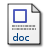# PERTIDAKSAMAAN AZUMA PADA MARTINGALE UNTUK MENENTUKAN SUPREMUM PELUANG

Sudarno, Sudarno (2008) PERTIDAKSAMAAN AZUMA PADA MARTINGALE UNTUK MENENTUKAN SUPREMUM PELUANG. Jurnal Matematika dan Komputer, 10 (2). pp. 66-72. ISSN 1410-8518Microsoft Word - Published Version345Kb

## Abstract

Counting probability a two-tailed hypothesis determine level of the significance. This case follows positive and negative random variables. So that the probability distribution is a symmetric. The probability will be counted by Azuma inequality on martingales. The lowest upper bound is a decay exponential function. It is determined in some a, n, m, and  value by a simulation. The conclusion of this paper is that the random variable value is higher than the probability value (supremum) is lower, vise versa. Therefore, Its property is same as the distribution function

Item Type: Article Markov Inequality, Martingale, Doob Martingale, Azuma Inequality. Q Science > Q Science (General) Faculty of Science and Mathematics > Department of Statistics 1859 INVALID USER 30 Nov 2009 10:18 30 Nov 2009 10:18

Repository Staff Only: item control page